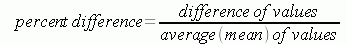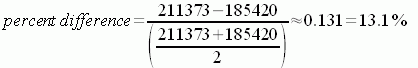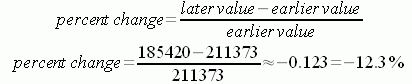SEARCH HOMEMath Central Quandaries & QueriesQuestion from Carolyn, a parent: What is the % difference between these two numbers 211373 & 185420Carolyn, we have several answers for you:

Hi there,

The % difference between two numbers is the absolute value of the difference between the two numbers, divided by the average of those two numbers, multiplied by 100%. That istherefore% difference = (approximately) 13.1%.

Notice that the (211373 - 185420) term is the difference between the two numbers, and the (211373 + 185420)/2 term is the average of the two numbers. This gives us a decimal that we then need to multiply by 100% to turn it into a percentage.

% difference is similar to but distinct from "% error ". % difference is used (for example) when comparing two independent measurements of the same quantity to find out how much the measurements differ. % error is used (for example) when comparing a single measurement of a quantity to the theoretical or "currently accepted" value of that quantity. In the case of % error , we would replace the average of the two terms in the denominator by the currently accepted value.

Hope this helps!
Gabriel.

Hi Carolyn.

I want to add a note to Gabe's response.

Percentage difference, percentage error and percentage change all ask for the difference of two numbers as a percentage of something. This phrase "of something" is always something we should think about when we talk about percentage. We should always ask "percentage of what?"

For percentage error where we know the actual value or the currently accepted value then we take the difference between the measurement and the actual value as a percentage of the actual value. This is what Gabe did.

For percentage change there is time involved. First you take a measurement and later you take a second measurement. You then evaluate the difference and represent this difference as a percentage of the starting value. So in particular if you had said that the value changed from 211373 to 185420 then the change is 185420 - 211373 = -25953. As a percentage of the starting value this is -25953/211373 * 100 = -12.28%. Since this is negative I could say there is a 12.28% decrease.

For percentage difference as in your question I agree with Gabe that you you should take the absolute value of the difference as a percentage of the average of the two values.

Penny.

Hi Carolyn.

Gabriel (the person who answered your question first) is a physicist. The term percent difference between two numbers doesn't have a really specific mathematical meaning, so hopefully the context you are using this for is the physical sciences.

Often, people are confused about percentages. Really, all they are is fractions (per-cent means per hundred, so 100 is the denominator: 13.1% = 13.1/100). And fractions are just ratios between two numbers: a numerator and a denominator.

So to have a "percent difference", I would look for two percentages (ratios) and take the difference of them as fractions. For example, the percent difference between 30% and 50% is 20%. But you don't have two ratios, you just have two large numbers.

Another aspect people ask about is percentage change. This is the change from an early value to a later value, and by convention, it is done with respect to the earlier value. What that means is that the earlier value is the denominator. You didn't say if your comparison was an earlier/later situation, but if 185420 was the later of the two, then you could say:So although you thought you had asked a rather simple question, Carolyn, the answer is rather long because percent difference isn't a mathematical term, but it is a scientific term. Only you can decide whether that fits the context of your question.

Stephen La Rocque. >Math Central is supported by the University of Regina and The Pacific Institute for the Mathematical Sciences.